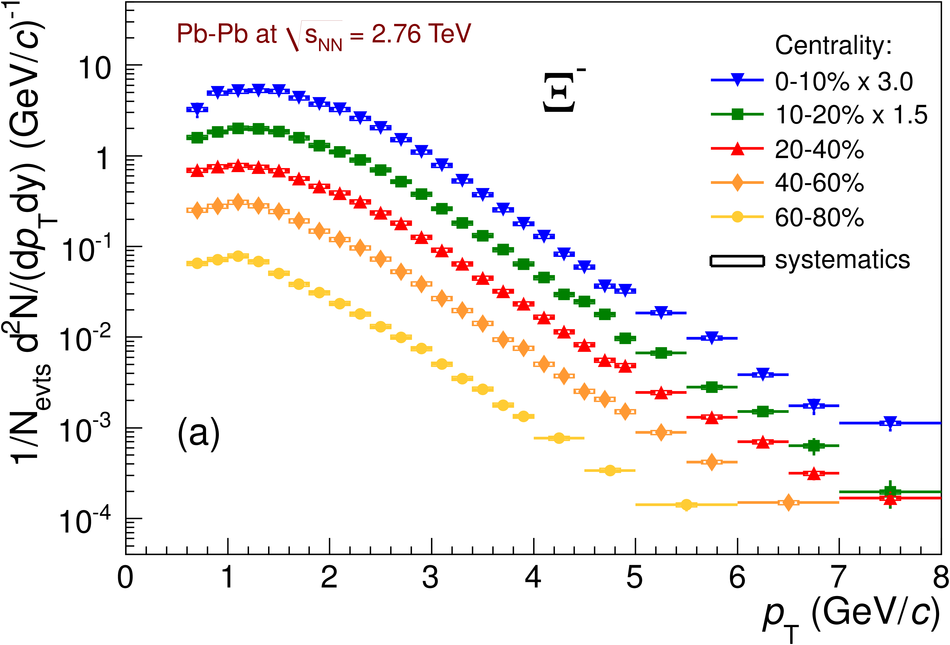# Figure 3

 Transverse momentum spectra for $\rm\Xi^{-}$ and $\rm\Omega^{-}$ (a,b) and their anti-particles (c,d) in five different centrality classes, from the most central (0-10%) to the most peripheral (60-80%) Pb-Pb collisions at $\snn=2.76$ TeV, for $|y|< 0.5$ at $\pt>1.8$ GeV/$c$ and $|y|< 0.3$ at $\pt< 1.8$ GeV/$c$. The statistical error bars are smaller than the symbols for most data points, while the systematic uncertainties are represented by the open boxes.